## Chapter 7: Correlation and Simple Linear Regression

In many studies, we measure more than one variable for each individual. For example, we measure precipitation and plant growth, or number of young with nesting habitat, or soil erosion and volume of water. We collect pairs of data and instead of examining each variable separately (univariate data), we want to find ways to describe bivariate data, in which two variables are measured on each subject in our sample. Given such data, we begin by determining if there is a relationship between these two variables. As the values of one variable change, do we see corresponding changes in the other variable?

We can describe the relationship between these two variables graphically and numerically. We begin by considering the concept of correlation.

Correlation is defined as the statistical association between two variables.

A correlation exists between two variables when one of them is related to the other in some way. A scatterplot is the best place to start. A scatterplot (or scatter diagram) is a graph of the paired (x, y) sample data with a horizontal x-axis and a vertical y-axis. Each individual (x, y) pair is plotted as a single point.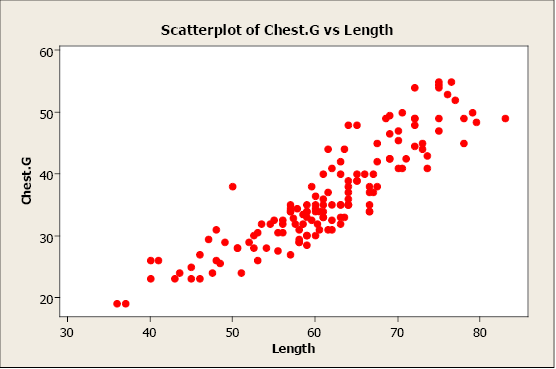Figure 1. Scatterplot of chest girth versus length.

In this example, we plot bear chest girth (y) against bear length (x). When examining a scatterplot, we should study the overall pattern of the plotted points. In this example, we see that the value for chest girth does tend to increase as the value of length increases. We can see an upward slope and a straight-line pattern in the plotted data points.

A scatterplot can identify several different types of relationships between two variables.

• A relationship has no correlation when the points on a scatterplot do not show any pattern.
• A relationship is non-linear when the points on a scatterplot follow a pattern but not a straight line.
• A relationship is linear when the points on a scatterplot follow a somewhat straight line pattern. This is the relationship that we will examine.

Linear relationships can be either positive or negative. Positive relationships have points that incline upwards to the right. As x values increase, y values increase. As x values decrease, y values decrease. For example, when studying plants, height typically increases as diameter increases.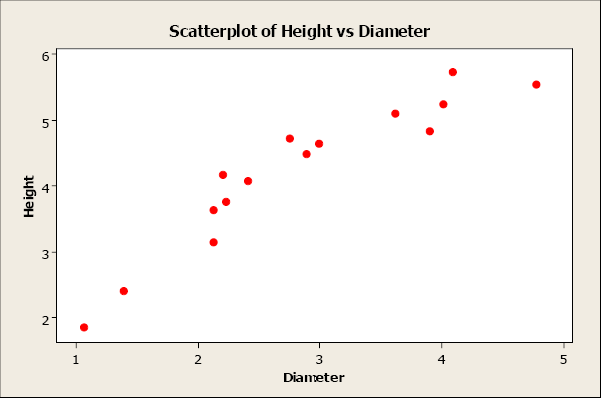Figure 2. Scatterplot of height versus diameter.

Negative relationships have points that decline downward to the right. As x values increase, y values decrease. As x values decrease, y values increase. For example, as wind speed increases, wind chill temperature decreases.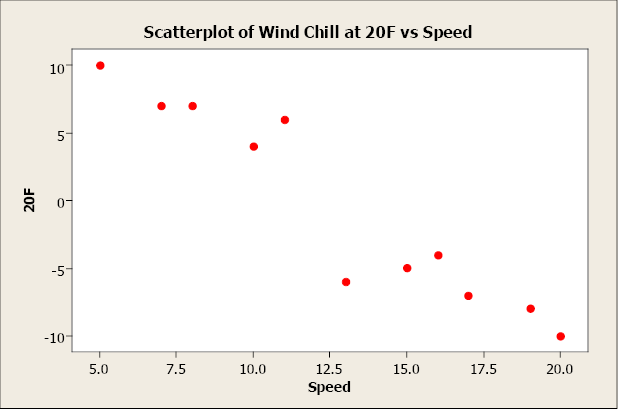Figure 3. Scatterplot of temperature versus wind speed.

Non-linear relationships have an apparent pattern, just not linear. For example, as age increases height increases up to a point then levels off after reaching a maximum height.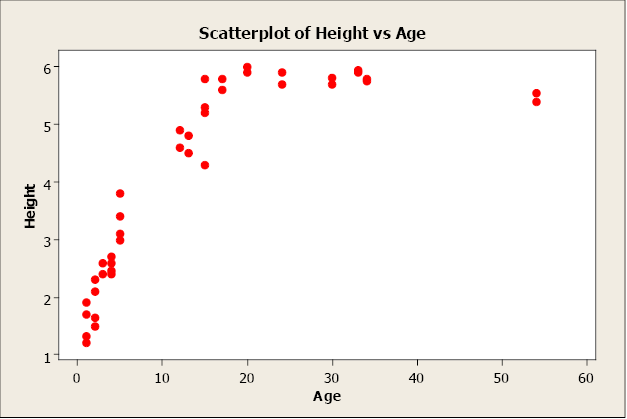Figure 4. Scatterplot of height versus age.

When two variables have no relationship, there is no straight-line relationship or non-linear relationship. When one variable changes, it does not influence the other variable.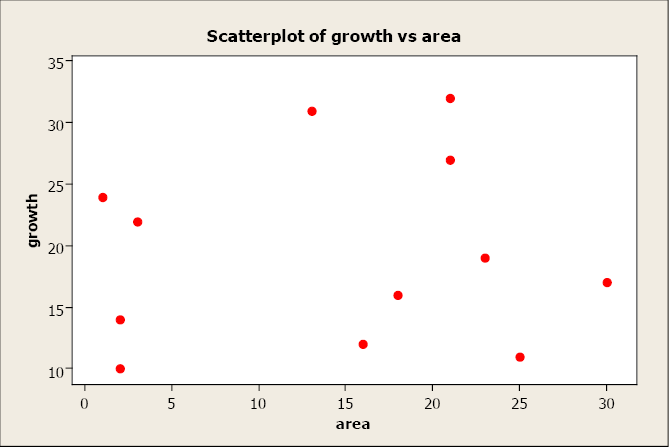Figure 5. Scatterplot of growth versus area.

## Linear Correlation Coefficient

Because visual examinations are largely subjective, we need a more precise and objective measure to define the correlation between the two variables. To quantify the strength and direction of the relationship between two variables, we use the linear correlation coefficient: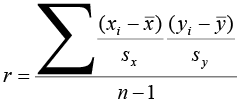where and sx are the sample mean and sample standard deviation of the x’s, and and sy are the mean and standard deviation of the y’s. The sample size is n.

An alternate computation of the correlation coefficient is: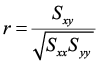where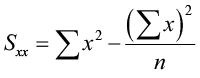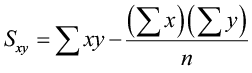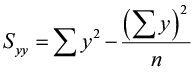The linear correlation coefficient is also referred to as Pearson’s product moment correlation coefficient in honor of Karl Pearson, who originally developed it. This statistic numerically describes how strong the straight-line or linear relationship is between the two variables and the direction, positive or negative.

The properties of “r”:

• It is always between -1 and +1.
• It is a unitless measure so “r” would be the same value whether you measured the two variables in pounds and inches or in grams and centimeters.
• Positive values of “r” are associated with positive relationships.
• Negative values of “r” are associated with negative relationships.

### Examples of Positive Correlation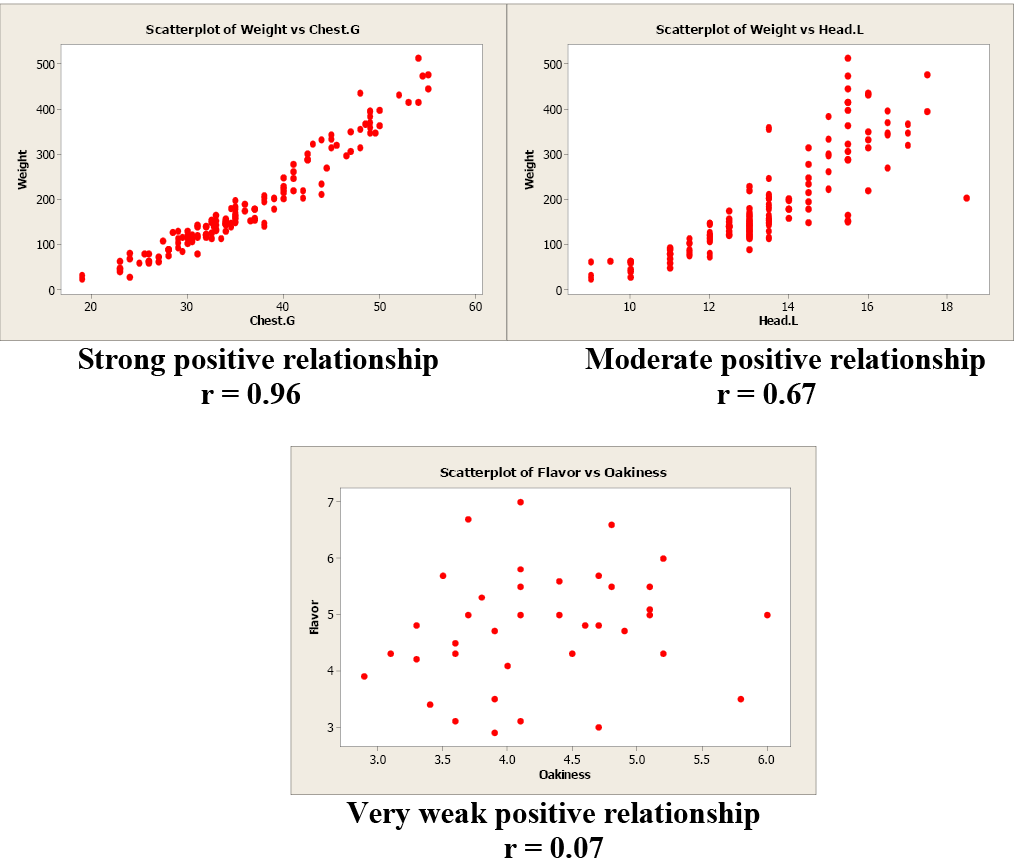Figure 6. Examples of positive correlation.

### Examples of Negative Correlation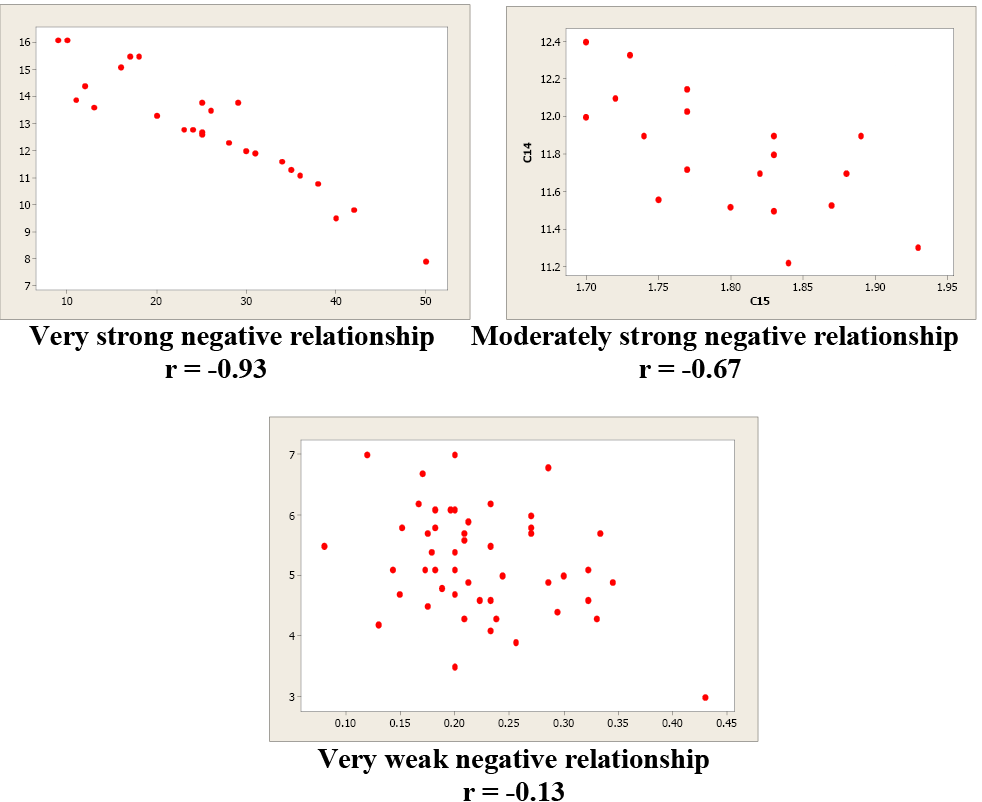Figure 7. Examples of negative correlation.

Correlation is not causation!!! Just because two variables are correlated does not mean that one variable causes another variable to change.

Examine these next two scatterplots. Both of these data sets have an r = 0.01, but they are very different. Plot 1 shows little linear relationship between x and y variables. Plot 2 shows a strong non-linear relationship. Pearson’s linear correlation coefficient only measures the strength and direction of a linear relationship. Ignoring the scatterplot could result in a serious mistake when describing the relationship between two variables.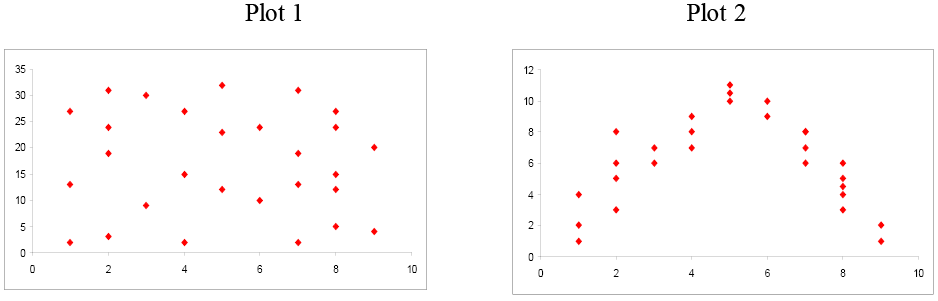Figure 8. Comparison of scatterplots.

When you investigate the relationship between two variables, always begin with a scatterplot. This graph allows you to look for patterns (both linear and non-linear). The next step is to quantitatively describe the strength and direction of the linear relationship using “r”. Once you have established that a linear relationship exists, you can take the next step in model building.

## Simple Linear Regression

Once we have identified two variables that are correlated, we would like to model this relationship. We want to use one variable as a predictor or explanatory variable to explain the other variable, the response or dependent variable. In order to do this, we need a good relationship between our two variables. The model can then be used to predict changes in our response variable. A strong relationship between the predictor variable and the response variable leads to a good model.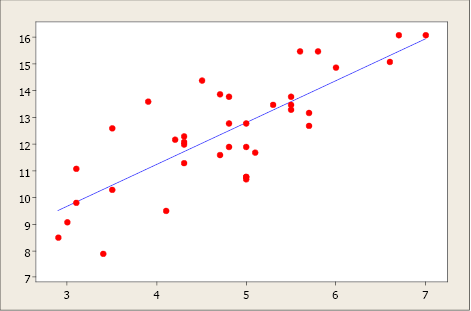Figure 9. Scatterplot with regression model.

A simple linear regression model is a mathematical equation that allows us to predict a response for a given predictor value.

Our model will take the form of = b 0 + b1x where b0 is the y-intercept, b1 is the slope, x is the predictor variable, and an estimate of the mean value of the response variable for any value of the predictor variable.

The y-intercept is the predicted value for the response (y) when x = 0. The slope describes the change in y for each one unit change in x. Let’s look at this example to clarify the interpretation of the slope and intercept.

### Example 1

A hydrologist creates a model to predict the volume flow for a stream at a bridge crossing with a predictor variable of daily rainfall in inches.

= 1.6 + 29x. The y-intercept of 1.6 can be interpreted this way: On a day with no rainfall, there will be 1.6 gal. of water/min. flowing in the stream at that bridge crossing. The slope tells us that if it rained one inch that day the flow in the stream would increase by an additional 29 gal./min. If it rained 2 inches that day, the flow would increase by an additional 58 gal./min.

### Example 2

What would be the average stream flow if it rained 0.45 inches that day?

= 1.6 + 29x = 1.6 + 29(0.45) = 14.65 gal./min.

The Least-Squares Regression Line (shortcut equations)

The equation is given by = b 0 + b1 x

where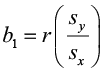is the slope and b0 = – b1 is the y-intercept of the regression line.

An alternate computational equation for slope is: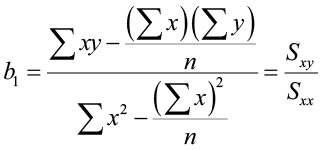This simple model is the line of best fit for our sample data. The regression line does not go through every point; instead it balances the difference between all data points and the straight-line model. The difference between the observed data value and the predicted value (the value on the straight line) is the error or residual. The criterion to determine the line that best describes the relation between two variables is based on the residuals.

Residual = Observed – Predicted

For example, if you wanted to predict the chest girth of a black bear given its weight, you could use the following model.

Chest girth = 13.2 +0.43 weight

The predicted chest girth of a bear that weighed 120 lb. is 64.8 in.

Chest girth = 13.2 + 0.43(120) = 64.8 in.

But a measured bear chest girth (observed value) for a bear that weighed 120 lb. was actually 62.1 in.

The residual would be 62.1 – 64.8 = -2.7 in.

A negative residual indicates that the model is over-predicting. A positive residual indicates that the model is under-predicting. In this instance, the model over-predicted the chest girth of a bear that actually weighed 120 lb.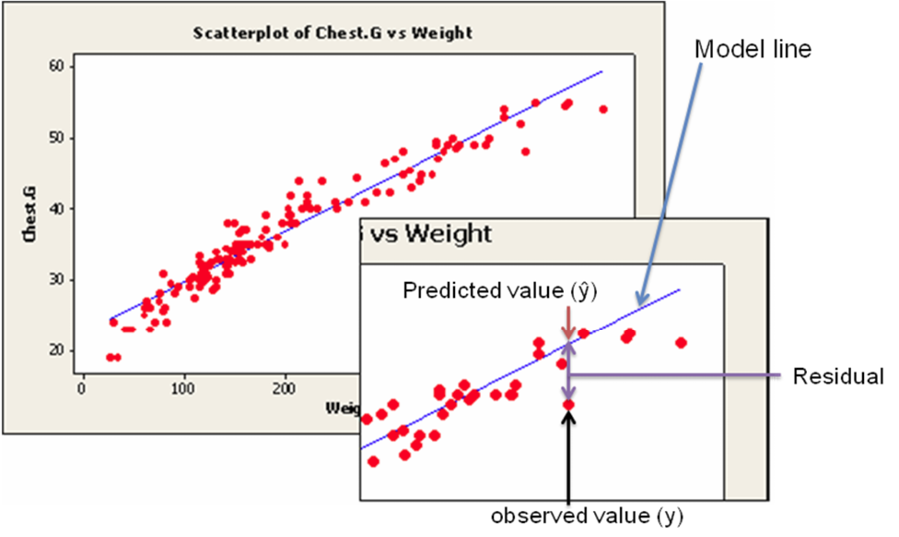Figure 10. Scatterplot with regression model illustrating a residual value.

This random error (residual) takes into account all unpredictable and unknown factors that are not included in the model. An ordinary least squares regression line minimizes the sum of the squared errors between the observed and predicted values to create a best fitting line. The differences between the observed and predicted values are squared to deal with the positive and negative differences.

## Coefficient of Determination

After we fit our regression line (compute b0 and b1), we usually wish to know how well the model fits our data. To determine this, we need to think back to the idea of analysis of variance. In ANOVA, we partitioned the variation using sums of squares so we could identify a treatment effect opposed to random variation that occurred in our data. The idea is the same for regression. We want to partition the total variability into two parts: the variation due to the regression and the variation due to random error. And we are again going to compute sums of squares to help us do this.

Suppose the total variability in the sample measurements about the sample mean is denoted by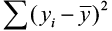, called the sums of squares of total variability about the mean (SST). The squared difference between the predicted value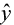and the sample mean is denoted by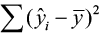, called the sums of squares due to regression (SSR). The SSR represents the variability explained by the regression line. Finally, the variability which cannot be explained by the regression line is called the sums of squares due to error (SSE) and is denoted by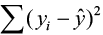. SSE is actually the squared residual.

 SST = SSR + SSE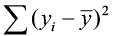=+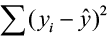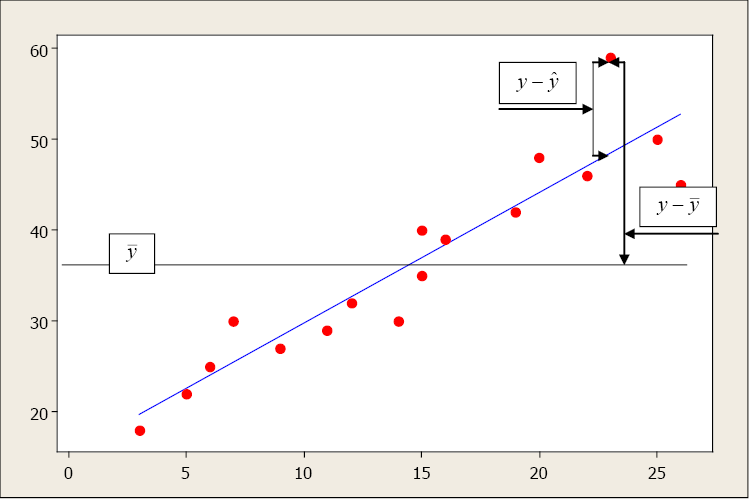Figure 11. An illustration of the relationship between the mean of the y’s and the predicted and observed value of a specific y.

The sums of squares and mean sums of squares (just like ANOVA) are typically presented in the regression analysis of variance table. The ratio of the mean sums of squares for the regression (MSR) and mean sums of squares for error (MSE) form an F-test statistic used to test the regression model.

The relationship between these sums of square is defined as

Total Variation = Explained Variation + Unexplained Variation

The larger the explained variation, the better the model is at prediction. The larger the unexplained variation, the worse the model is at prediction. A quantitative measure of the explanatory power of a model is R2, the Coefficient of Determination: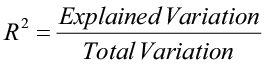The Coefficient of Determination measures the percent variation in the response variable (y) that is explained by the model.

• Values range from 0 to 1.
• An R2 close to zero indicates a model with very little explanatory power.
• An R2 close to one indicates a model with more explanatory power.

The Coefficient of Determination and the linear correlation coefficient are related mathematically.

R2 = r2

However, they have two very different meanings: r is a measure of the strength and direction of a linear relationship between two variables; R2 describes the percent variation in “y” that is explained by the model.

## Residual and Normal Probability Plots

Even though you have determined, using a scatterplot, correlation coefficient and R2, that x is useful in predicting the value of y, the results of a regression analysis are valid only when the data satisfy the necessary regression assumptions.

1. The response variable (y) is a random variable while the predictor variable (x) is assumed non-random or fixed and measured without error.
2. The relationship between y and x must be linear, given by the model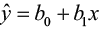.
3. The error of random term the values ε are independent, have a mean of 0 and a common variance σ2, independent of x, and are normally distributed.

We can use residual plots to check for a constant variance, as well as to make sure that the linear model is in fact adequate. A residual plot is a scatterplot of the residual (= observed – predicted values) versus the predicted or fitted (as used in the residual plot) value. The center horizontal axis is set at zero. One property of the residuals is that they sum to zero and have a mean of zero. A residual plot should be free of any patterns and the residuals should appear as a random scatter of points about zero.

A residual plot with no appearance of any patterns indicates that the model assumptions are satisfied for these data.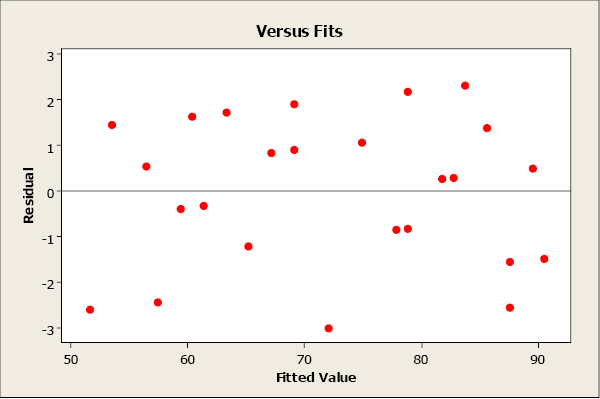Figure 12. A residual plot.

A residual plot that has a “fan shape” indicates a heterogeneous variance (non-constant variance). The residuals tend to fan out or fan in as error variance increases or decreases.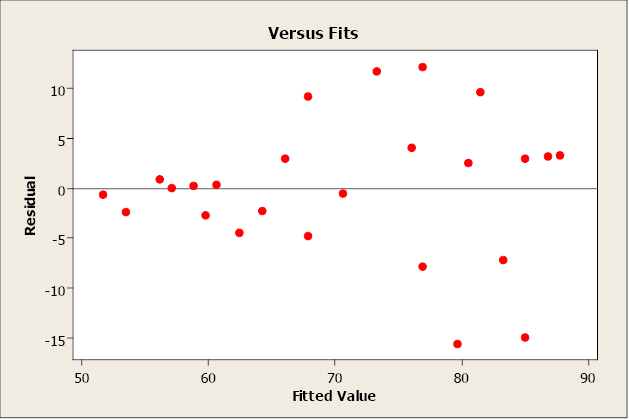Figure 13. A residual plot that indicates a non-constant variance.

A residual plot that tends to “swoop” indicates that a linear model may not be appropriate. The model may need higher-order terms of x, or a non-linear model may be needed to better describe the relationship between y and x. Transformations on x or y may also be considered.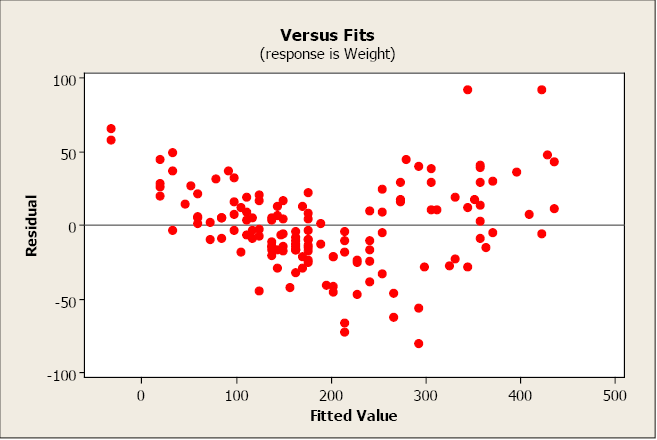Figure 14. A residual plot that indicates the need for a higher order model.

A normal probability plot allows us to check that the errors are normally distributed. It plots the residuals against the expected value of the residual as if it had come from a normal distribution. Recall that when the residuals are normally distributed, they will follow a straight-line pattern, sloping upward.

This plot is not unusual and does not indicate any non-normality with the residuals.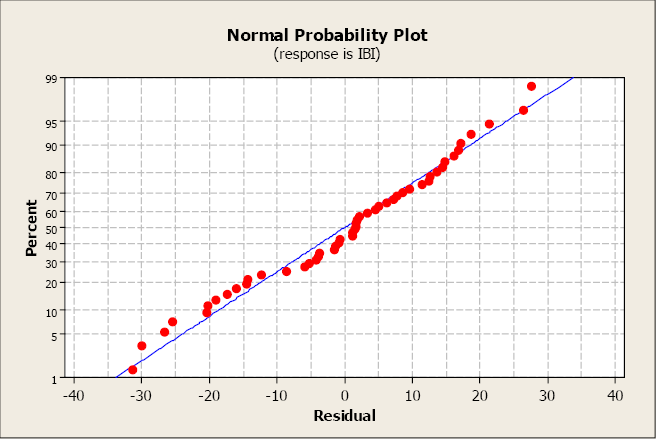Figure 15. A normal probability plot.

This next plot clearly illustrates a non-normal distribution of the residuals.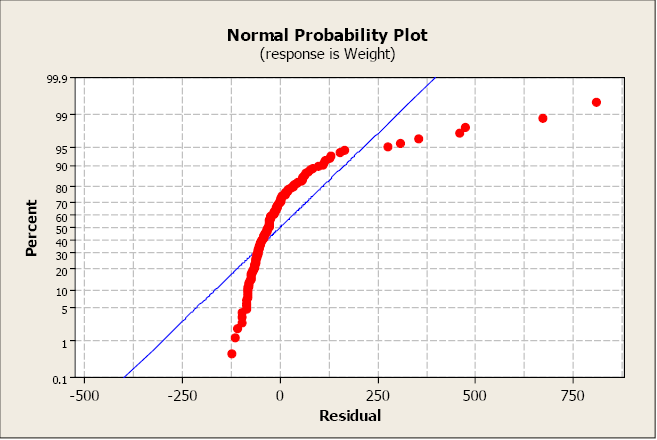Figure 16. A normal probability plot, which illustrates non-normal distribution.

The most serious violations of normality usually appear in the tails of the distribution because this is where the normal distribution differs most from other types of distributions with a similar mean and spread. Curvature in either or both ends of a normal probability plot is indicative of nonnormality.

## Population Model

Our regression model is based on a sample of n bivariate observations drawn from a larger population of measurements.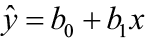We use the means and standard deviations of our sample data to compute the slope (b1) and y-intercept (b0) in order to create an ordinary least-squares regression line. But we want to describe the relationship between y and x in the population, not just within our sample data. We want to construct a population model. Now we will think of the least-squares line computed from a sample as an estimate of the true regression line for the population.

The Population Model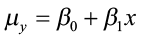, where μy is the population mean response, β0 is the y-intercept, and β1 is the slope for the population model.

In our population, there could be many different responses for a value of x. In simple linear regression, the model assumes that for each value of x the observed values of the response variable y are normally distributed with a mean that depends on x. We use μy to represent these means. We also assume that these means all lie on a straight line when plotted against x (a line of means).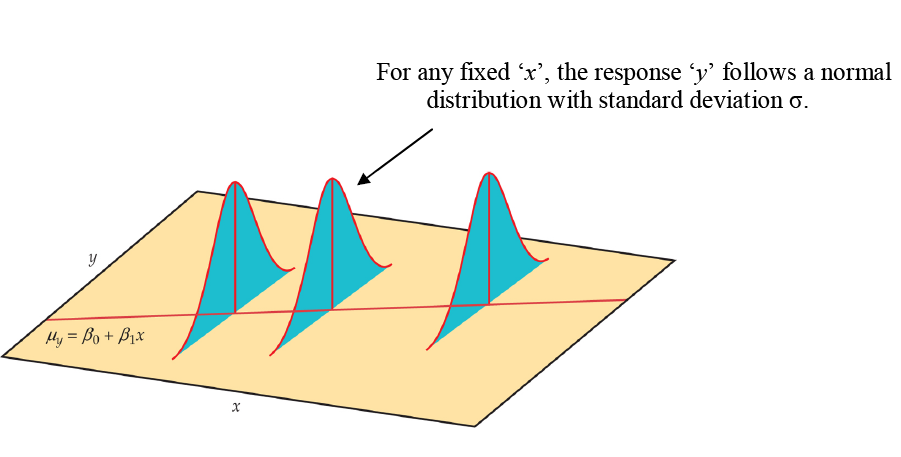Figure 17. The statistical model for linear regression; the mean response is a straight-line function of the predictor variable.

The sample data then fit the statistical model:

Data = fit + residual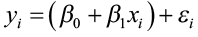where the errors (εi) are independent and normally distributed N (0, σ). Linear regression also assumes equal variance of y (σ is the same for all values of x). We use ε (Greek epsilon) to stand for the residual part of the statistical model. A response y is the sum of its mean and chance deviation ε from the mean. The deviations ε represents the “noise” in the data. In other words, the noise is the variation in y due to other causes that prevent the observed (x, y) from forming a perfectly straight line.

The sample data used for regression are the observed values of y and x. The response y to a given x is a random variable, and the regression model describes the mean and standard deviation of this random variable y. The intercept β0, slope β1, and standard deviation σ of y are the unknown parameters of the regression model and must be estimated from the sample data.

• The value of from the least squares regression line is really a prediction of the mean value of y (μy) for a given value of x.
• The least squares regression line (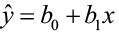) obtained from sample data is the best estimate of the true population regression line
(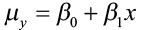).

is an unbiased estimate for the mean response μy
b0 is an unbiased estimate for the intercept β0
b1 is an unbiased estimate for the slope β1

## Parameter Estimation

Once we have estimates of β0 and β1 (from our sample data b0 and b1), the linear relationship determines the estimates of μy for all values of x in our population, not just for the observed values of x. We now want to use the least-squares line as a basis for inference about a population from which our sample was drawn.

Model assumptions tell us that b0 and b1 are normally distributed with means β0 and β1 with standard deviations that can be estimated from the data. Procedures for inference about the population regression line will be similar to those described in the previous chapter for means. As always, it is important to examine the data for outliers and influential observations.

In order to do this, we need to estimate σ, the regression standard error. This is the standard deviation of the model errors. It measures the variation of y about the population regression line. We will use the residuals to compute this value. Remember, the predicted value of y () for a specific x is the point on the regression line. It is the unbiased estimate of the mean response (μy) for that x. The residual is:

residual = observed – predicted

ei = yi =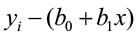The residual ei corresponds to model deviation εi where Σ ei = 0 with a mean of 0. The regression standard error s is an unbiased estimate of σ.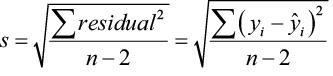The quantity s is the estimate of the regression standard error (σ) and s2 is often called the mean square error (MSE). A small value of s suggests that observed values of y fall close to the true regression line and the lineshould provide accurate estimates and predictions.

## Confidence Intervals and Significance Tests for Model Parameters

In an earlier chapter, we constructed confidence intervals and did significance tests for the population parameter μ (the population mean). We relied on sample statistics such as the mean and standard deviation for point estimates, margins of errors, and test statistics. Inference for the population parameters β0 (slope) and β1 (y-intercept) is very similar.

Inference for the slope and intercept are based on the normal distribution using the estimates b0 and b1. The standard deviations of these estimates are multiples of σ, the population regression standard error. Remember, we estimate σ with s (the variability of the data about the regression line). Because we use s, we rely on the student t-distribution with (n – 2) degrees of freedom.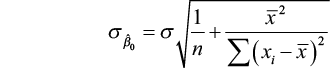The standard error for estimate of β0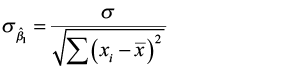The standard error for estimate of β1

We can construct confidence intervals for the regression slope and intercept in much the same way as we did when estimating the population mean.

A confidence interval for β0 : b0 ± t α/2 SEb0

A confidence interval for β1 : b1 ± t α/2 SEb1

where SEb0 and SEb1 are the standard errors for the y-intercept and slope, respectively.

We can also test the hypothesis H0: β1 = 0. When we substitute β1 = 0 in the model, the x-term drops out and we are left with μy = β0. This tells us that the mean of y does NOT vary with x. In other words, there is no straight line relationship between x and y and the regression of y on x is of no value for predicting y.

Hypothesis test for β1

H0: β1 =0

H1: β1 0

The test statistic is t = b1 / SEb1

We can also use the F-statistic (MSR/MSE) in the regression ANOVA table*

*Recall that t2 = F

So let’s pull all of this together in an example.

### Example 3

The index of biotic integrity (IBI) is a measure of water quality in streams. As a manager for the natural resources in this region, you must monitor, track, and predict changes in water quality. You want to create a simple linear regression model that will allow you to predict changes in IBI in forested area. The following table conveys sample data from a coastal forest region and gives the data for IBI and forested area in square kilometers. Let forest area be the predictor variable (x) and IBI be the response variable (y).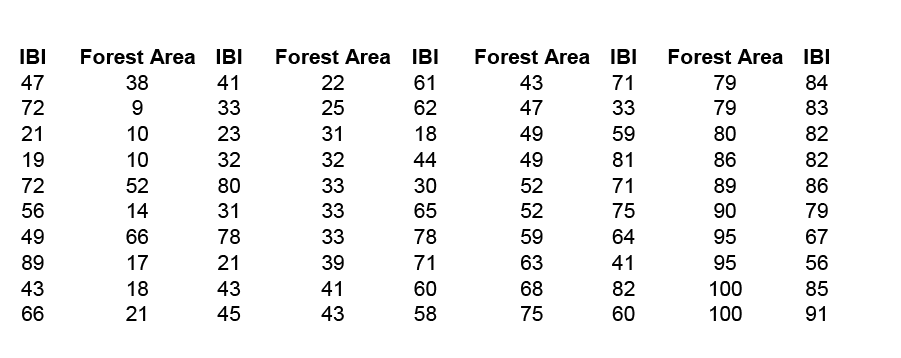Table 1. Observed data of biotic integrity and forest area.

We begin with a computing descriptive statistics and a scatterplot of IBI against Forest Area.

= 47.42; sx 27.37; = 58.80; sy = 21.38; r = 0.735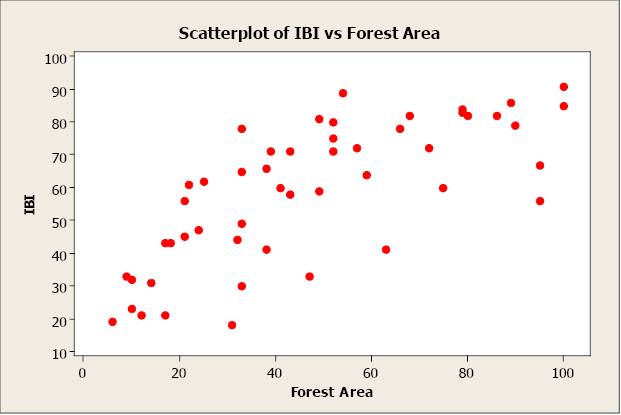Figure 18. Scatterplot of IBI vs. Forest Area.

There appears to be a positive linear relationship between the two variables. The linear correlation coefficient is r = 0.735. This indicates a strong, positive, linear relationship. In other words, forest area is a good predictor of IBI. Now let’s create a simple linear regression model using forest area to predict IBI (response).

First, we will compute b0 and b1 using the shortcut equations.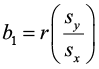=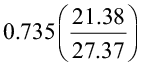=0.574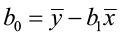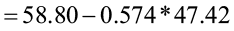= 31.581

The regression equation is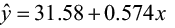.

Now let’s use Minitab to compute the regression model. The output appears below.

#### Regression Analysis: IBI versus Forest Area

The regression equation is IBI = 31.6 + 0.574 Forest Area

 Predictor Coef SE Coef T P Constant 31.583 4.177 7.56 0.000 Forest Area 0.57396 0.07648 7.50 0.000 S = 14.6505 R-Sq = 54.0% R-Sq(adj) = 53.0%
 Analysis of Variance Source DF SS MS F P Regression 1 12089 12089 56.32 0.000 Residual Error 48 10303 215 Total 49 22392

The estimates for β0 and β1 are 31.6 and 0.574, respectively. We can interpret the y-intercept to mean that when there is zero forested area, the IBI will equal 31.6. For each additional square kilometer of forested area added, the IBI will increase by 0.574 units.

The coefficient of determination, R2, is 54.0%. This means that 54% of the variation in IBI is explained by this model. Approximately 46% of the variation in IBI is due to other factors or random variation. We would like R2 to be as high as possible (maximum value of 100%).

The residual and normal probability plots do not indicate any problems.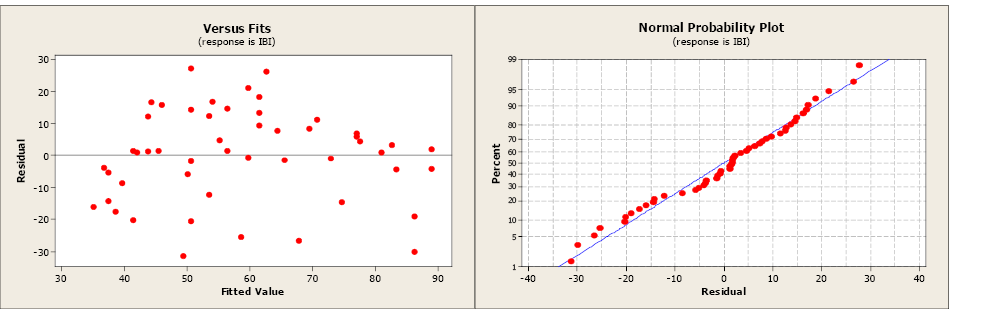Figure 19. A residual and normal probability plot.

The estimate of σ, the regression standard error, is s = 14.6505. This is a measure of the variation of the observed values about the population regression line. We would like this value to be as small as possible. The MSE is equal to 215. Remember, the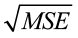= s. The standard errors for the coefficients are 4.177 for the y-intercept and 0.07648 for the slope.

We know that the values b0 = 31.6 and b1 = 0.574 are sample estimates of the true, but unknown, population parameters β0 and β1. We can construct 95% confidence intervals to better estimate these parameters. The critical value (tα/2) comes from the student t-distribution with (n – 2) degrees of freedom. Our sample size is 50 so we would have 48 degrees of freedom. The closest table value is 2.009.

95% confidence intervals for β0 and β1

b0 ± tα/2 SEb0 = 31.6 ± 2.009(4.177) = (23.21, 39.99)

b1 ± tα/2 SEb1 = 0.574 ± 2.009(0.07648) = (0.4204, 0.7277)

The next step is to test that the slope is significantly different from zero using a 5% level of significance.

 H0: β1 =0 H1: β1 ≠0

t = b1 / SEb1 = 0.574/0.07648 = 7.50523

We have 48 degrees of freedom and the closest critical value from the student t-distribution is 2.009. The test statistic is greater than the critical value, so we will reject the null hypothesis. The slope is significantly different from zero. We have found a statistically significant relationship between Forest Area and IBI.

The Minitab output also report the test statistic and p-value for this test.

 The regression equation is IBI = 31.6 + 0.574 Forest Area Predictor Coef SE Coef T P Constant 31.583 4.177 7.56 0.000 Forest Area 0.57396 0.07648 7.50 0.000 S = 14.6505 R-Sq = 54.0% R-Sq(adj) = 53.0%
 Analysis of Variance Source DF SS MS F P Regression 1 12089 12089 56.32 0.000 Residual Error 48 10303 215 Total 49 22392

The t test statistic is 7.50 with an associated p-value of 0.000. The p-value is less than the level of significance (5%) so we will reject the null hypothesis. The slope is significantly different from zero. The same result can be found from the F-test statistic of 56.32 (7.5052 = 56.32). The p-value is the same (0.000) as the conclusion.

## Confidence Interval for μy

Now that we have created a regression model built on a significant relationship between the predictor variable and the response variable, we are ready to use the model for

• estimating the average value of y for a given value of x
• predicting a particular value of y for a given value of x

Let’s examine the first option. The sample data of n pairs that was drawn from a population was used to compute the regression coefficients b0 and b1 for our model, and gives us the average value of y for a specific value of x through our population model. For every specific value of x, there is an average y (μy), which falls on the straight line equation (a line of means). Remember, that there can be many different observed values of the y for a particular x, and these values are assumed to have a normal distribution with a mean equal to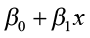and a variance of σ2. Since the computed values of b0 and b1 vary from sample to sample, each new sample may produce a slightly different regression equation. Each new model can be used to estimate a value of y for a value of x. How far will our estimatorbe from the true population mean for that value of x? This depends, as always, on the variability in our estimator, measured by the standard error.

It can be shown that the estimated value of y when x = x0 (some specified value of x), is an unbiased estimator of the population mean, and that is normally distributed with a standard error of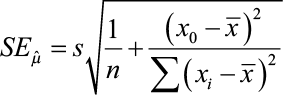We can construct a confidence interval to better estimate this parameter (μy) following the same procedure illustrated previously in this chapter.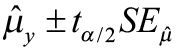where the critical value tα/2 comes from the student t-table with (n – 2) degrees of freedom.

Statistical software, such as Minitab, will compute the confidence intervals for you. Using the data from the previous example, we will use Minitab to compute the 95% confidence interval for the mean response for an average forested area of 32 km.

 Predicted Values for New Observations New Obs Fit SE Fit 95% CI 1 49.9496 2.38400 (45.1562,54.7429)

If you sampled many areas that averaged 32 km. of forested area, your estimate of the average IBI would be from 45.1562 to 54.7429.

You can repeat this process many times for several different values of x and plot the confidence intervals for the mean response.

 x 95% CI 20 (37.13, 48.88) 40 (50.22, 58.86) 60 (61.43, 70.61) 80 (70.98, 84.02) 100 (79.88, 98.07)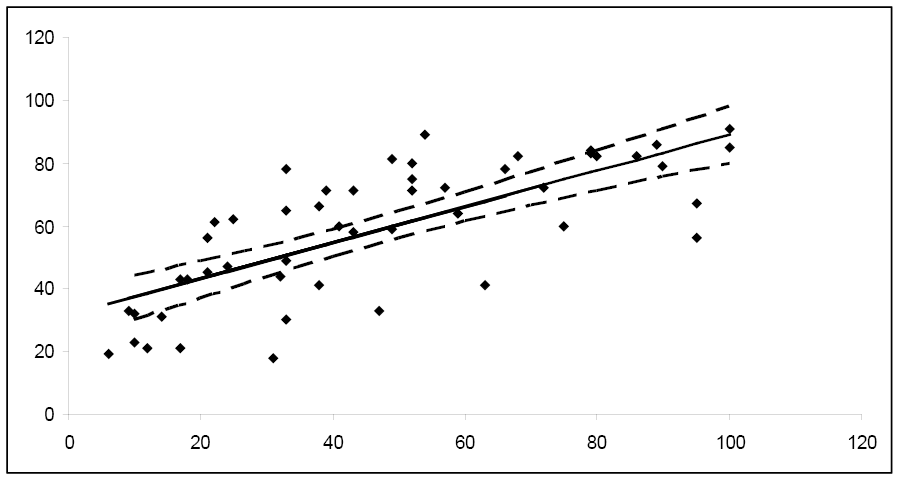Figure 20. 95% confidence intervals for the mean response.

Notice how the width of the 95% confidence interval varies for the different values of x. Since the confidence interval width is narrower for the central values of x, it follows that μy is estimated more precisely for values of x in this area. As you move towards the extreme limits of the data, the width of the intervals increases, indicating that it would be unwise to extrapolate beyond the limits of the data used to create this model.

## Prediction Intervals

What if you want to predict a particular value of y when x = x0? Or, perhaps you want to predict the next measurement for a given value of x? This problem differs from constructing a confidence interval for μy. Instead of constructing a confidence interval to estimate a population parameter, we need to construct a prediction interval. Choosing to predict a particular value of y incurs some additional error in the prediction because of the deviation of y from the line of means. Examine the figure below. You can see that the error in prediction has two components:

1. The error in using the fitted line to estimate the line of means
2. The error caused by the deviation of y from the line of means, measured by σ2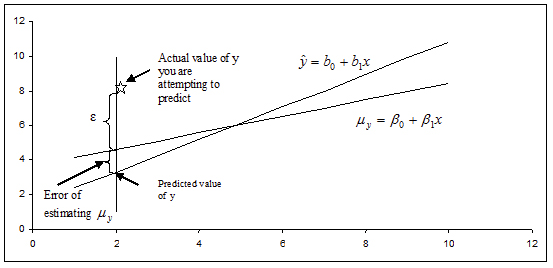Figure 21. Illustrating the two components in the error of prediction.

The variance of the difference between y and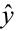is the sum of these two variances and forms the basis for the standard error of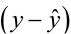used for prediction. The resulting form of a prediction interval is as follows: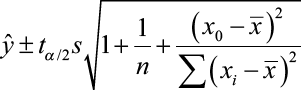where x0 is the given value for the predictor variable, n is the number of observations, and tα/2 is the critical value with (n – 2) degrees of freedom.

Software, such as Minitab, can compute the prediction intervals. Using the data from the previous example, we will use Minitab to compute the 95% prediction interval for the IBI of a specific forested area of 32 km.

 Predicted Values for New Observations New Obs Fit SE Fit 95% PI 1 49.9496 2.38400 (20.1053, 79.7939)

You can repeat this process many times for several different values of x and plot the prediction intervals for the mean response.

 x 95% PI 20 (13.01, 73.11) 40 (24.77, 84.31) 60 (36.21, 95.83) 80 (47.33, 107.67) 100 (58.15, 119.81)

Notice that the prediction interval bands are wider than the corresponding confidence interval bands, reflecting the fact that we are predicting the value of a random variable rather than estimating a population parameter. We would expect predictions for an individual value to be more variable than estimates of an average value.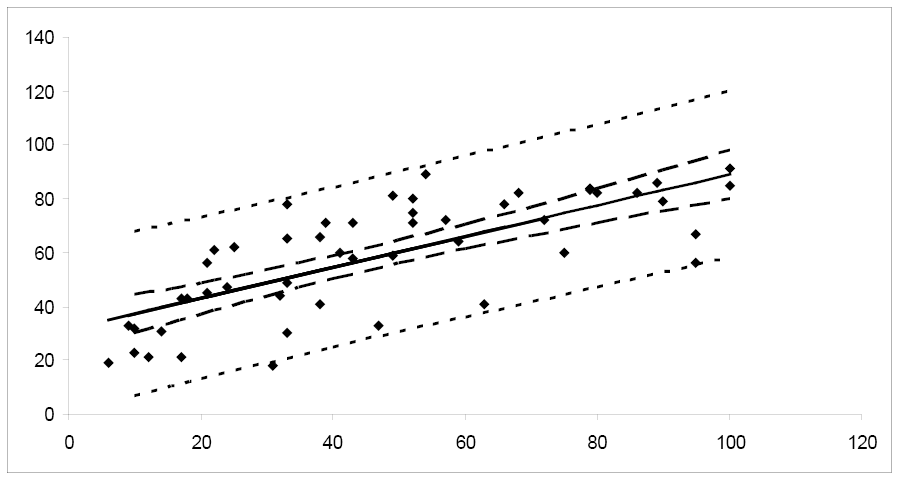Figure 22. A comparison of confidence and prediction intervals.

## Transformations to Linearize Data Relationships

In many situations, the relationship between x and y is non-linear. In order to simplify the underlying model, we can transform or convert either x or y or both to result in a more linear relationship. There are many common transformations such as logarithmic and reciprocal. Including higher order terms on x may also help to linearize the relationship between x and y. Shown below are some common shapes of scatterplots and possible choices for transformations. However, the choice of transformation is frequently more a matter of trial and error than set rules.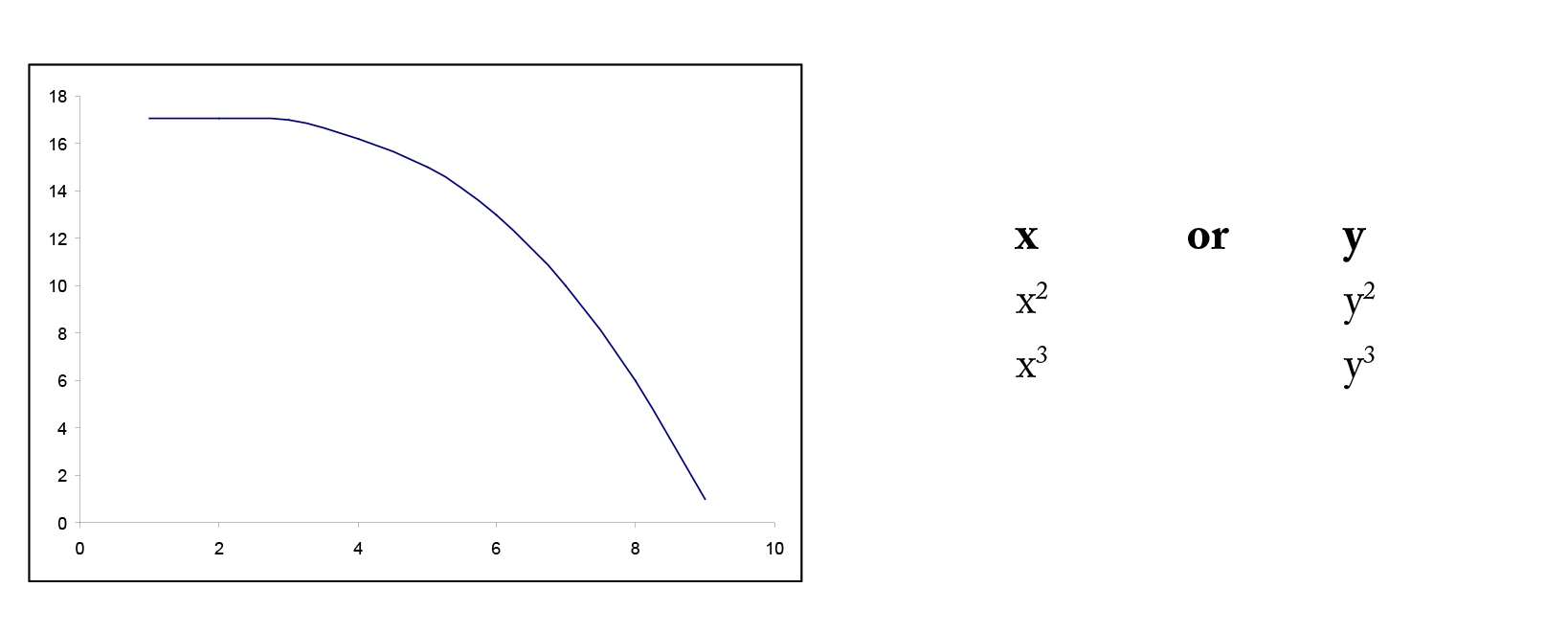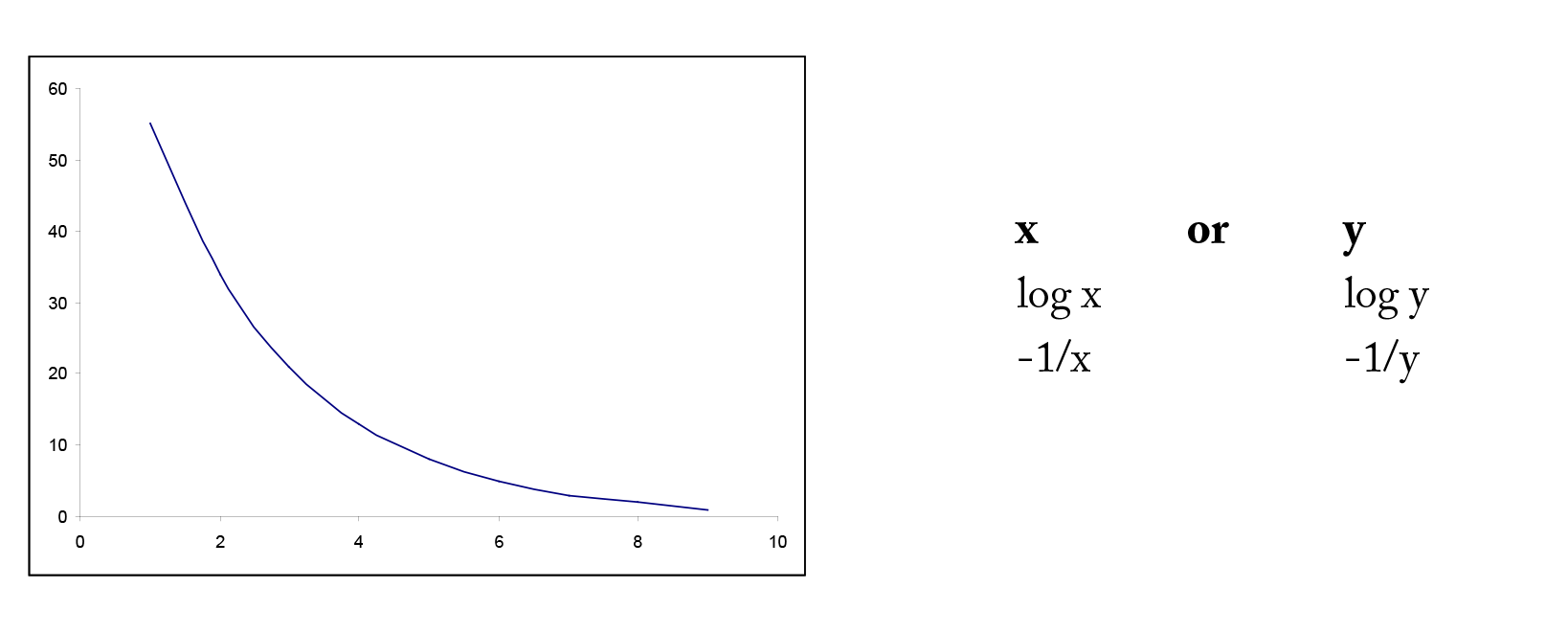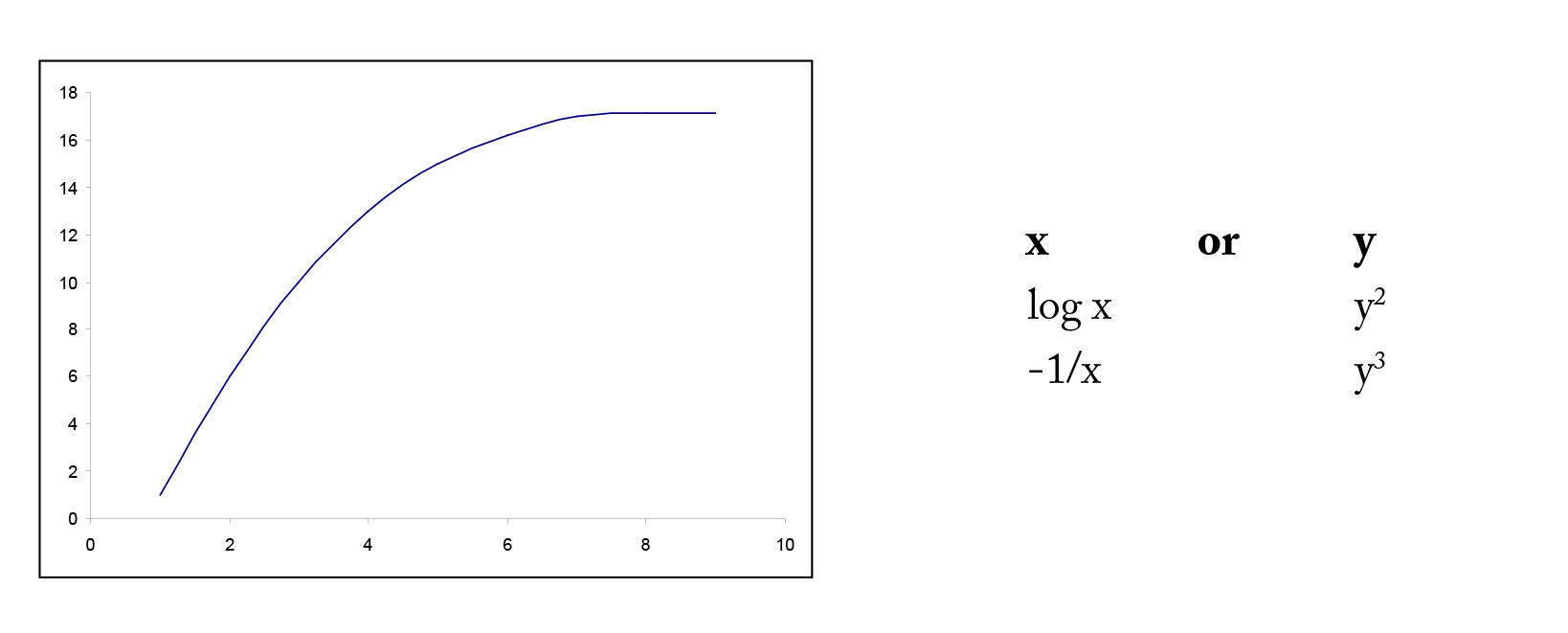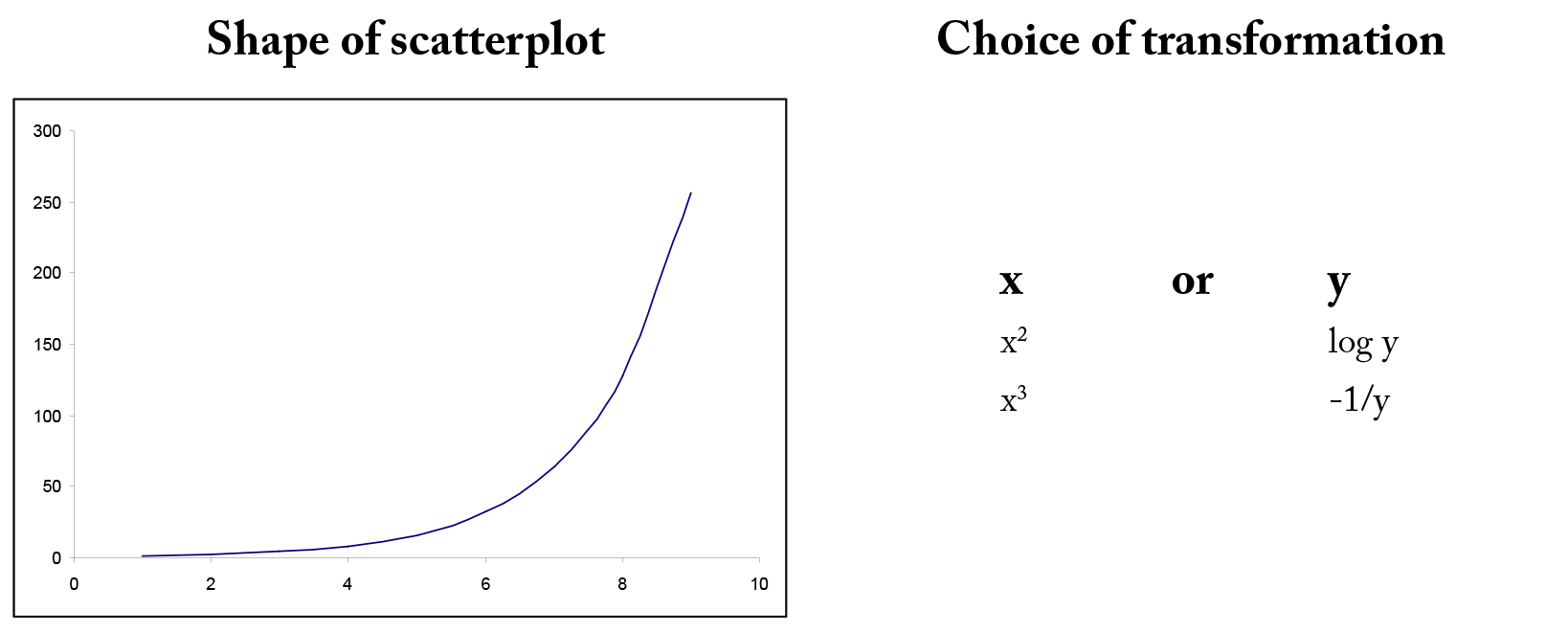Figure 23. Examples of possible transformations for x and y variables.

### Example 4

A forester needs to create a simple linear regression model to predict tree volume using diameter-at-breast height (dbh) for sugar maple trees. He collects dbh and volume for 236 sugar maple trees and plots volume versus dbh. Given below is the scatterplot, correlation coefficient, and regression output from Minitab.Figure 24. Scatterplot of volume versus dbh.

Pearson’s linear correlation coefficient is 0.894, which indicates a strong, positive, linear relationship. However, the scatterplot shows a distinct nonlinear relationship.

#### Regression Analysis: volume versus dbh

 The regression equation is volume = – 51.1 + 7.15 dbh Predictor Coef SE Coef T P Constant -51.097 3.271 -15.62 0.000 dbh 7.1500 0.2342 30.53 0.000 S = 19.5820 R-Sq = 79.9% R-Sq(adj) = 79.8%
 Analysis of Variance Source DF SS MS F P Regression 1 357397 357397 932.04 0.000 Residual Error 234 89728 383 Total 235 447125

The R2 is 79.9% indicating a fairly strong model and the slope is significantly different from zero. However, both the residual plot and the residual normal probability plot indicate serious problems with this model. A transformation may help to create a more linear relationship between volume and dbh.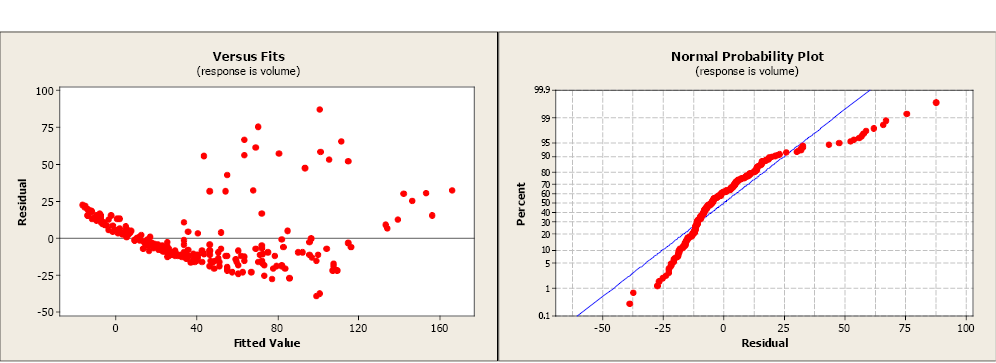Figure 25. Residual and normal probability plots.

Volume was transformed to the natural log of volume and plotted against dbh (see scatterplot below). Unfortunately, this did little to improve the linearity of this relationship. The forester then took the natural log transformation of dbh. The scatterplot of the natural log of volume versus the natural log of dbh indicated a more linear relationship between these two variables. The linear correlation coefficient is 0.954.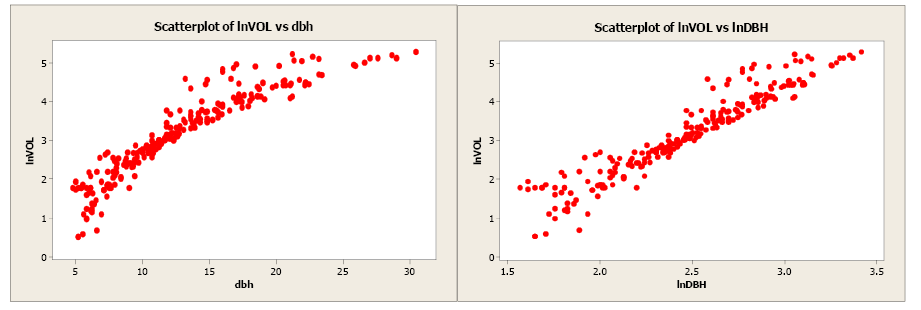Figure 26. Scatterplots of natural log of volume versus dbh and natural log of volume versus natural log of dbh.

The regression analysis output from Minitab is given below.

#### Regression Analysis: lnVOL vs. lnDBH

 The regression equation is lnVOL = – 2.86 + 2.44 lnDBH Predictor Coef SE Coef T P Constant -2.8571 0.1253 -22.80 0.000 lnDBH 2.44383 0.05007 48.80 0.000 S = 0.327327 R-Sq = 91.1% R-Sq(adj) = 91.0%
 Analysis of Variance Source DF SS MS F P Regression 1 255.19 255.19 2381.78 0.000 Residual Error 234 25.07 0.11 Total 235 280.26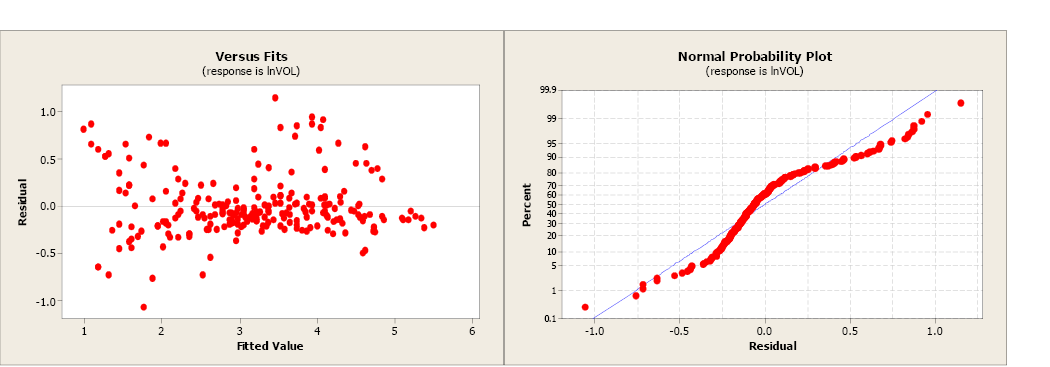Figure 27. Residual and normal probability plots.

The model using the transformed values of volume and dbh has a more linear relationship and a more positive correlation coefficient. The slope is significantly different from zero and the R2 has increased from 79.9% to 91.1%. The residual plot shows a more random pattern and the normal probability plot shows some improvement.

There are many possible transformation combinations possible to linearize data. Each situation is unique and the user may need to try several alternatives before selecting the best transformation for x or y or both.

## Software Solutions

### Minitab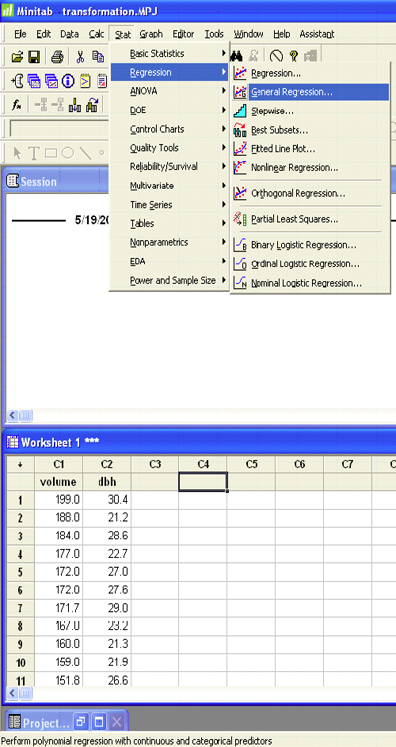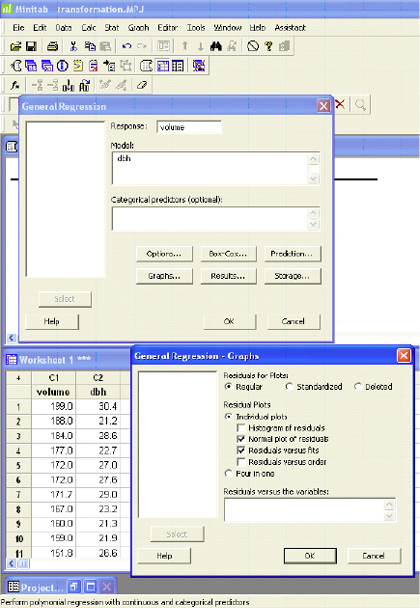The Minitab output is shown above in Ex. 4.

### Excel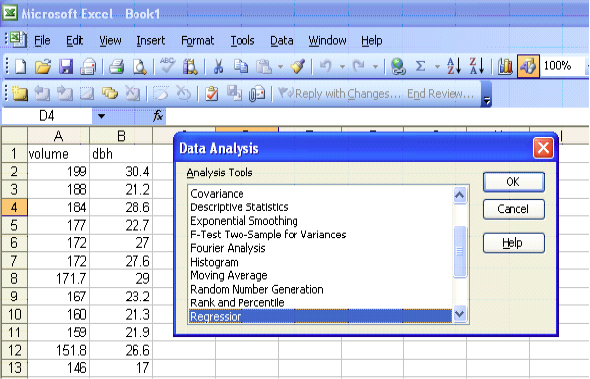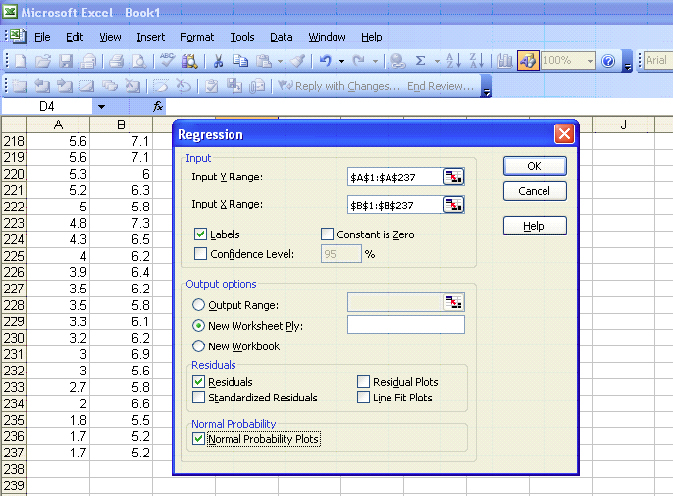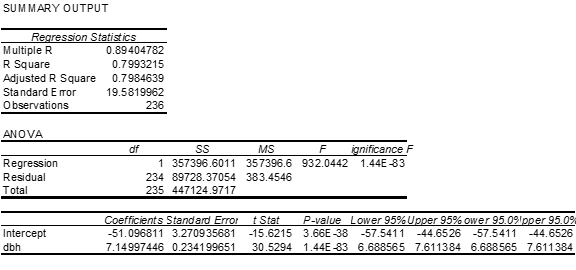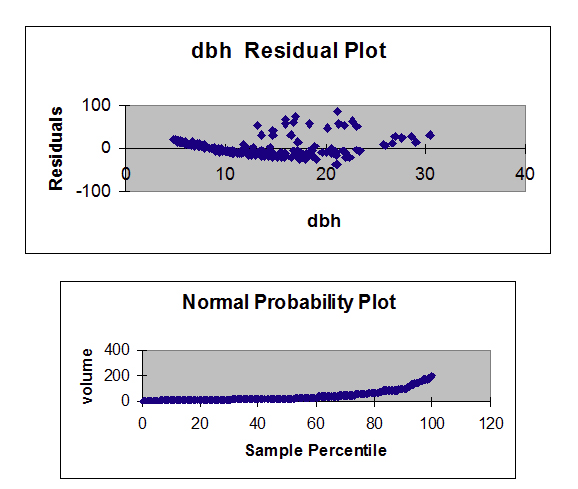Figure 28. Residual and normal probability plots.